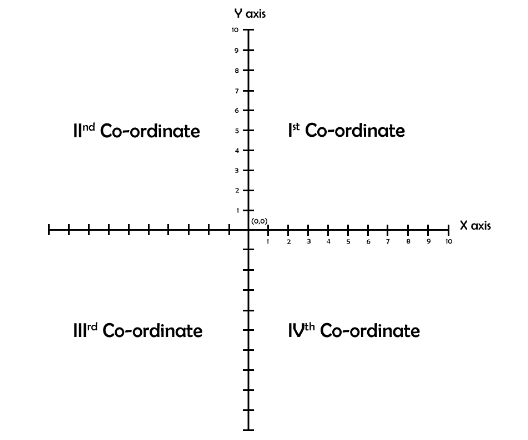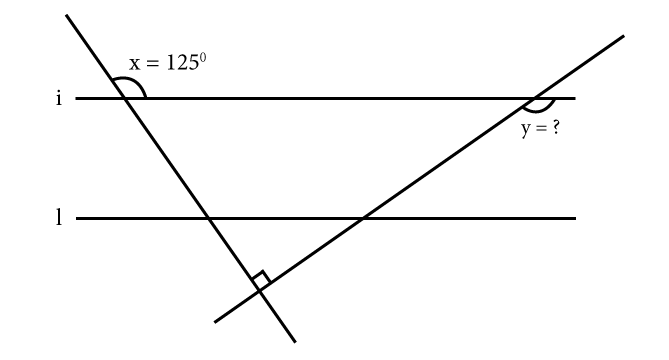# GRE Quantitative: Algebra – Coordinate Geometry

Coordinate Geometry is the third most liked topic in the Quantitative Reasoning section of GRE. Usually the questions asked in coordinate geometry hold high weightage and because of that a single right solution in this topic can benefit you a lot. So, let us check the basic concepts of coordinate geometry and tackle it on the day of your GRE exam with full confidence.

Two perpendicular real number lines that intersect each other at their respective zero points defines the rectangular coordinate system, which is commonly known as the x and y- coordinate plane or xy plane. In the x and y plane, the horizontal line is known as the x- axis, whereas, the vertical line is y- axis. The point where these two lines intersect is known as the origin, and it is denoted by O. In the x- axis, the values represented on the right of zero are positive values, whereas, values on the left of zero are negative values. Meanwhile, the y- axis is also divided into positive and negative segment. The first half of y- axis that are represented above 0 are positive whereas points below zero are negative.

These perpendicular intersecting lines divide the plane into four quadrants, which can be represented as:-In this figure,

• The values in the first quadrant, both x and y, are positive
• The values in the second quadrant, y is positive whereas x is negative.
• The values in the third quadrant, both x and y is negative.
• The values in the fourth quadrant, x is positive whereas y is negative.

Representation is x, y- coordinates:

The value is represented inside a bracket where the first value represents the x-coordinate and second value represents the y-coordinate.

The representation of values in all four quadrants is as follows:

• (x, y) ← First quadrant
• (-x, y) ← Second quadrant
• (-x, -y) ← Third Quadrant
• (x, -y) ← Fourth quadrant

Let’s solve a problem to understand it in detail.

Question: Here, lines i and l are parallelFind the value of y.

Solution: By using the complementary and supplementary rules of geometry, as the lines i and l are parallel to one another and the sum of all angles of a triangle is 180o.

Therefore,

• Step 1: 180o – 125o = 55o

We can notice that a right-angled triangle is formed with the line i acting as the hypotenuse. As we have the angle adjacent to the x=125o angle, which is 55o , we can calculate the third angle inside the right-angled triangle.

• Step 2: 55o + 90o + z = 180o
• Step 3:z = 35o

Now that we have the value of the third angle, we can use it to calculate the value of y using the straight line property.

• Step 4: z + y = 180o
35o + y = 180o
y = 145o

BYJU’S will be glad to help you in your GRE preparation journey. You can ask for any assistance related to GRE from us by just giving a missed call at +918884544444, or you can drop an SMS. You can write to us at gre@byjus.com.